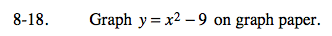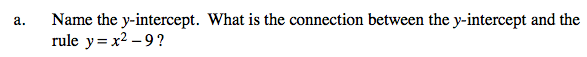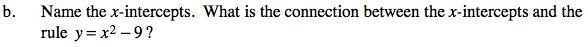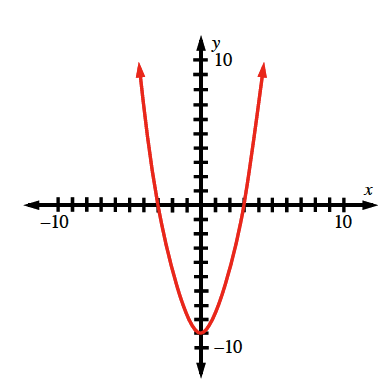### Home > CAAC > Chapter 8 > Lesson 8.1.2 > Problem8-18

8-18.The y-intercept is where the parabola intersects the
y-axis.

The y-intercept is where x = 0.
y = (0)² − 9
y = −9

The x-intercept is where the parabola intersects the
x-axis.

The x-intercept is where y = 0
0 = x² − 9
9 = x²

x = −3, +3

${\sqrt{9} = x}$